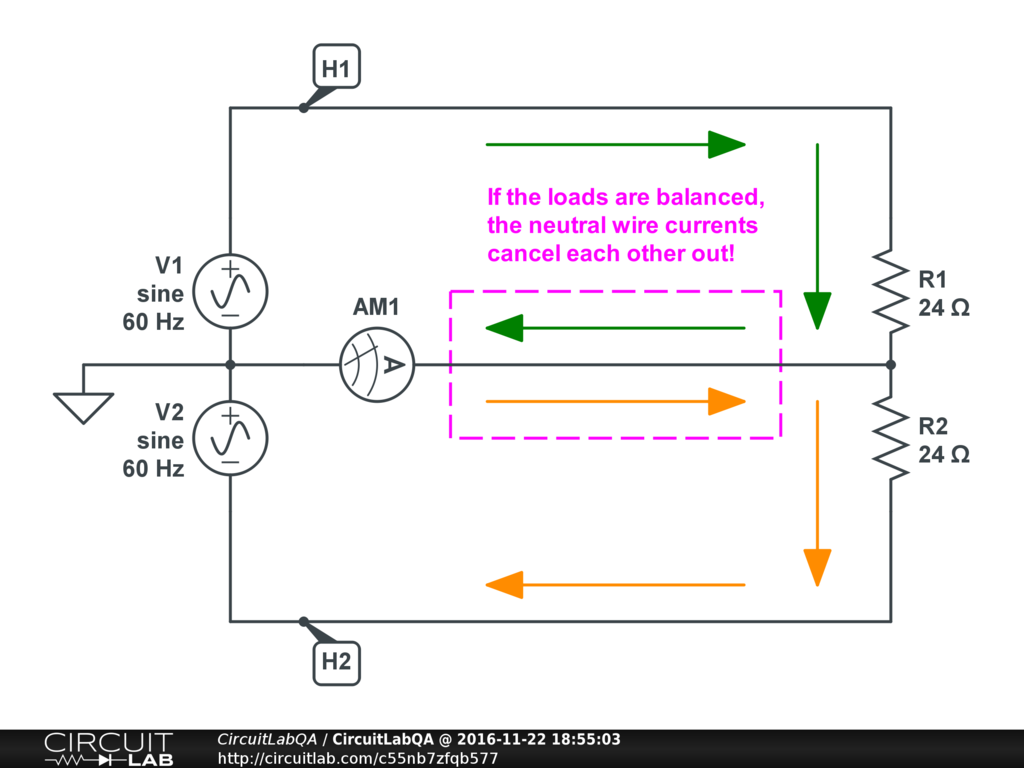## Generator extension cord thick enough

 I have a 2 kilowatt diesel generator in case it's a rough winter. Never needed it knock on wood but like to be ready. Anyway I went down to Home Depot and got a new cord for it so I can keep it further from the house because its so loud. They sold me a 25ft 10/4 cord, says 30 amp, 250 volt on it. So heres what I don't get. If I do 20 amps on the first phase, 20 amps on the second phase, doesn't the neutral wire have to be twice as thick to carry 40 amps? by CAMIE538 November 21, 2016 A 2Kw generator will pull max 9 amps at 220 v total (W=V*A) or 9A * 110V * 2 therefore the wire you seleted is way over the requirements. The neutral only let 20 amps through when using 10a per 110v branches but when using 220v, the current does not flow through the neutral. by c_dupre November 22, 2016

 Actually since we're talking about single phase 240V AC power (not 3 phase) coming from a small generator, there are four wires: hot #1 [BLACK wire] = $V_{H1} (t) = 120 \sqrt {2} \times sin(2 \pi * 60 t)$ hot #2 [RED wire] = $V_{H2} (t) = -120 \sqrt {2} \times sin(2 \pi * 60 t)$ neutral [WHITE wire] = $V_{NEUTRAL} = 0$ ground [GREEN wire] = $V_{GND} = 0$ (Let's not worry about the difference between ground and neutral; grounding is worthy of its own question.) If you have a 240V load, you'd connect it between two hot wires: $$V_{H1} (t) - V_{H2} (t) = 240 \sqrt {2} \times sin(2 \pi * 60 t)$$ But if you have a 120V load, you'd connect it either between hot #1 and neutral: $$V_{H1} (t) - V_{NEUTRAL} = 120 \sqrt {2} \times sin(2 \pi * 60 t)$$ or between hot #2 and neutral: $$V_{H2} (t) - V_{NEUTRAL} = -120 \sqrt {2} \times sin(2 \pi * 60 t)$$ To your lamp or motor or whatever, it doesn't matter that there's a minus sign -- it's really just saying it's 180 degrees out of phase. Ideally you want to balance your 120V loads between both sides of the circuit, so roughly half the load is on hot #1, and roughly half on hot #2. Here's why: if you have 5 amps on hot #1, and 5 amps on hot #2, you might think that, since currents add up, you must be sending 10 amps down the neutral line. But in reality, since this is AC, there's a phase component too -- and (ideally, at least) the two currents are 180 degrees out of phase. So just as $V_{H1}$ is at its peak maximum, sending lots of current though its load and into the neutral wire, at the same moment $V_{H2}$ is at its negative peak, pulling lots of current out of the neutral wire! So in reality the currents (mostly) cancel out:I think the green and orange arrows kind of show what's happening here at a moment in time. Run the simulation if you're not sure... If the loads are unbalanced on the two hot wires, the neutral wire only carries enough current to make up for the imbalance. So, in fact, in 3-phase systems, the neutral wire can actually be thinner than the phase wires. +1 vote by mrobbins November 22, 2016

 When the snow is twenty foot deep it will muffle the generator noise and you won't notice it over the screaming of the blizzard and the creaking and groaning of the roof timbers. But, yes, what goes down one live wire comes back up the other and vice versa. The neutral, as its name implies, sees very little. Don't forget to fit an RCD at the generator end, you don't want to 'cute the livestock. +1 vote by mikerogerswsm December 04, 2016

CircuitLab's Q&A site is a FREE questions and answers forum for electronics and electrical engineering students, hobbyists, and professionals.

We encourage you to use our built-in schematic & simulation software to add more detail to your questions and answers.

Acceptable Questions:

• Concept or theory questions
• Practical engineering questions
• “Homework” questions
• Software/hardware intersection
• Best practices
• Design choices & component selection
• Troubleshooting

Unacceptable Questions:

• Non-English language content
• Non-question discussion
• Non-electronics questions
• Vendor-specific topics
• Pure software questions
• CircuitLab software support

Please respect that there are both seasoned experts and total newbies here: please be nice, be constructive, and be specific!